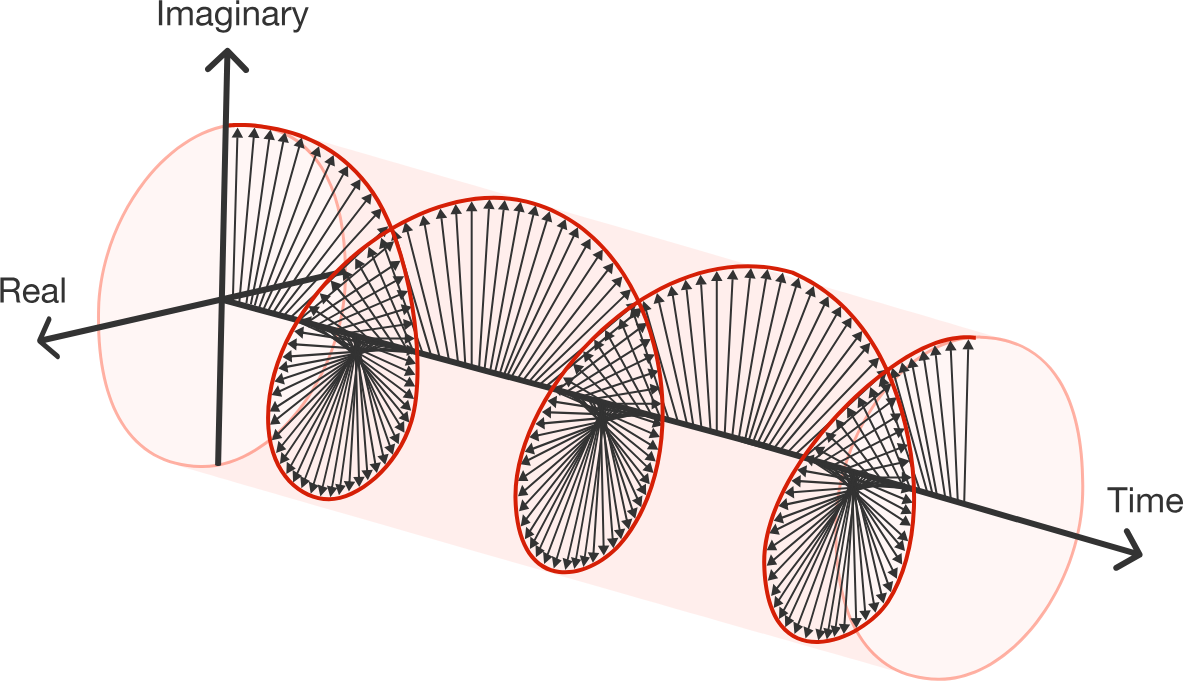# The Path To Euler's Formula

$e^ {i\pi} +1 = 0$

In Euler's identity, which value is not actually a number (real or complex)?

Euler's identity combines the five values: $$e, i, \pi,$$ 1, and 0 in an elegant and entirely non-obvious way. There is something beautiful and powerful going on here, and our goal in this course is for you to come to fully understand it!

This introduction quiz covers just the tip of the iceberg that is Euler's formula, just enough so that you can start to get an idea of why it's interesting and how it can be useful.

# The Path To Euler's Formula

What does $$e^{i\pi}$$ mean?

When most math students (even those who have seen the imaginary constant $$i$$ before) first run into Euler’s identity, their initial response is typically something like:

• First 2 seconds: “Wow, it’s so small and simple looking.”

• Next 2 seconds: “...and it combines so many important constants so elegantly.”

• Next 4 years-lifetime: “...wait. That actually makes no sense whatsoever. It’s like saying the square root of five apples is equal to blue--it’s just completely nonsensical.”

# The Path To Euler's Formula

But Euler’s formula is not mystical. For the most part, it works like any other algebra you may have encountered. For example, exponential rules like the power rule still apply.

True or False?

$e^{2\pi i} - 1 = 0$

Hint: Take a look at the proof attempt below. Do you believe it, or does it contain a mistake?

1. $$e^{\pi i} + 1 = 0$$.

2. Therefore, $$e^{\pi i} = -1$$.

3. Therefore, $$(e^{\pi i})^2 = (-1)^2$$.

4. Therefore, $$e^{2\pi i} = 1$$.

Euler’s formula is just as elegant with $$\tau$$ as it is with $$\pi.$$ $$(\tau = 2\pi$$ and is pronounced “tau.”$$)$$

# The Path To Euler's Formula

Euler's full equation is $e^{i\theta} = \cos(\theta) + i \sin(\theta),$ of which Euler’s identity, $$e^{i\pi} = -1,$$ is just one case where $$\theta = \pi:$$ $e^{i\pi} = \cos(\pi) + i\sin(\pi) = -1.$

Using this fact as a hint, what is the value of $$\sin(\pi) ?$$

Looking at the equation above, it shouldn't be too surprising that, in order to really understand Euler's formula, you'll need to build up a very high level of confidence working with

• complex numbers
• functions and transformations
• exponents
• trigonometry.

# The Path To Euler's Formula

Using Euler’s formula, you’ll be able to derive the most annoying of the trigonometry identities in seconds instead of needing to memorize them!

Below is an example of using Euler’s full formula to derive two trig identities at the same time.

Based on the math below, which of the answer options is a correct trig identity?

$e^{\theta i} = \cos(\theta) + i \sin(\theta)$

1. $$e^{2\theta i} = \cos(2\theta) + i \sin(2\theta)$$

2. $$\big(e^{\theta i}\big)^2 = \big(\cos(\theta) + i\sin(\theta)\big)^2$$

3. Since $$\big(e^{\theta i}\big)^2 = e^{2\theta i}$$, $$\big(\cos(\theta) + i\sin(\theta)\big)^2 = \cos(2\theta) + i\sin(2\theta)$$.

4. Expanding the left hand side gives $$\cos^2(\theta) - \sin^2(\theta)+ 2i\cos(\theta)\sin(\theta)$$
$$= \cos(2\theta) + i\sin(2\theta)$$.

Hint: Remember that, if two complex numbers are equal, then their real and imaginary parts must both be equal.

# The Path To Euler's Formula

In this course, you’ll also learn to apply Euler’s formula to some geometry/trigonometry that you may have seen before, and also to some physics that you probably haven’t seen before.Not only is Euler’s formula beautiful, it’s also an incredibly powerful tool!

Ready to get started?

×